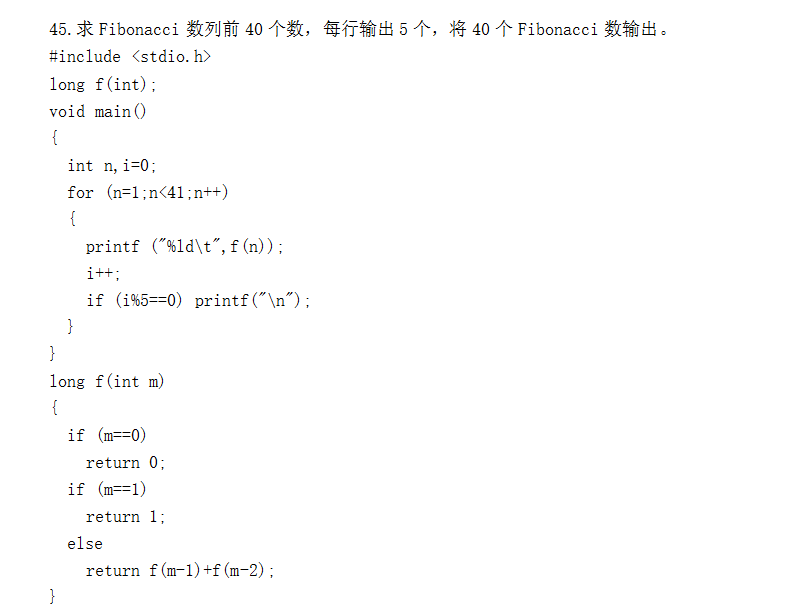return +递归函数，这种的

1个回答

``````long f1(int m, int i, int a, int b);

long f(int m)
{
if (m < 3) return 1;
return f1(m, 3, 1, 1);
}

long f1(int m, int i, int a, int b)
{
if (i > m) return b;
b = a + b;
a = b - a;
return f1(m, i + 1,  a, b);
}
``````

``````long f(int m)
{
int a = 1;
int b = 1;
for (int i = 3; i <= m; i++)
{
b = a + b;
a = b - a;
}
return b;
}
``````